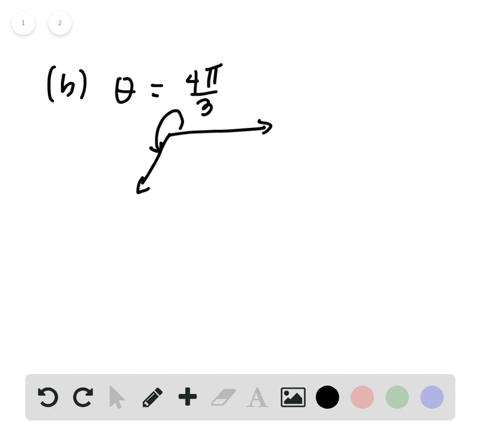🎉 The Study-to-Win Winning Ticket number has been announced! Go to your Tickets dashboard to see if you won! 🎉View Winning Ticket### Coterminal Angles in Radians In Exercises 7 and 8…

01:22University of Southern California
Problem 6

# Coterminal Angles in Degrees In Exercises 5 and 6 , determine two coterminal angles in degree measure (one positive and one negative) for each angle.

## Discussion

You must be signed in to discuss.

## Video Transcript

problem Number six asks us to determine to co terminal angles in degree measures one positive and one negative for each of the following two angles. Now a really simple way to tackle these kinds of problems is to just keep in mind that when we look at this angle that they've set up for us, thes to raise cannot move it all right. They need to have the same orientation relation to each other. And we can maintain the orientation by taking advantage of the fact that a circle is 360 degrees. So we can add 360 degrees to this measure and the rays will be in the same position or he can subtract 360 degrees will still be in the same position, but that effectively different. So for a if we want to find a positive angle measure that is co terminal, we can take 300 degrees plus 360 degrees and get 660 degrees. So that be number one. Another thing we can do is take our 300 degrees and subtract 360 degrees, and that will give us negative 60. And so these two angles 660 degrees and negative. 60 are both co terminal with 300 degrees now for B. We're just gonna do the exact same thing trying to co terminal angles by adding or subtracting, you know, 360 degrees. So let's add 360 did for number for letter B first so negative for 20 plus 360 that's gonna equal negative 60 degrees, which, you know, right off the bat lets us know that all of these angles air co terminal with these angles. But let's find a new one that's different from these two. So I'm going to subtract 360 from or 20 hoops. Let me reset room quick, minus 360 equals minus 7 80 All right. And so Oh, well, there were other negative in that case, so I guess we'll just have 360 of this one. Ha ha ha. And we'll see. Oh, wow, 300 degrees. It's go terminal with this one. But, um, negative 780 is also co terminal with B. And so yeah, that's how you do it